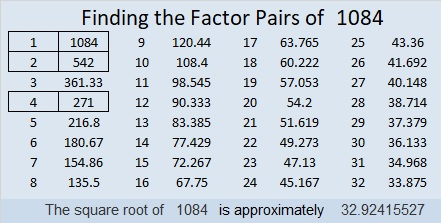# 1084 and Level 4

Where should you put all the numbers 1 to 12 in both the top row and the first column?  You will have to think about it and use logic. Some of the clues might be tricky, but you’ll figure it all out.Print the puzzles or type the solution in this excel file: 12 factors 1080-1086

Here are some facts about the number 1084:

• 1084 is a composite number.
• Prime factorization: 1084 = 2 × 2 × 271, which can be written 1084 = 2² × 271
• The exponents in the prime factorization are 1 and 2. Adding one to each and multiplying we get (2 + 1)(1 + 1) = 3 × 2  = 6. Therefore 1084 has exactly 6 factors.
• Factors of 1084: 1, 2, 4, 271, 542, 1084
• Factor pairs: 1084 = 1 × 1084, 2 × 542, or 4 × 271
• Taking the factor pair with the largest square number factor, we get √1084 = (√4)(√271) = 2√271 ≈ 32.924161084 is the sum of the 18 prime numbers from 23 to 101.

It is also the sum of six consecutive prime numbers:
167 + 173 + 179 + 181 + 191 + 193  = 1084

This site uses Akismet to reduce spam. Learn how your comment data is processed.